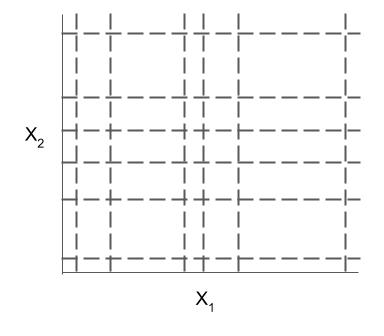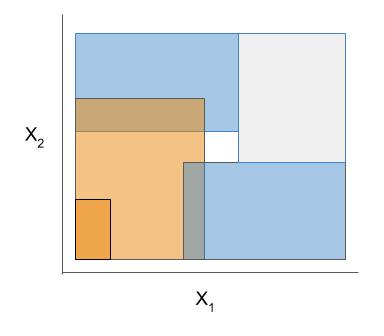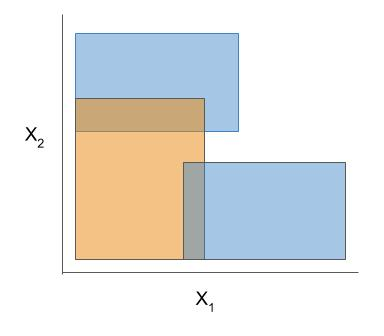Interpretable ML package 🔍 for concise, transparent, and accurate predictive modeling (sklearn-compatible).Chandan Singh e614c0bf37 add downloads badge 1 day ago
.github a1aa4ac8b8 update readme 2 weeks ago
docs 70b3197cb5 RuleFitClassifier docs update 4 days ago
imodels cba6377a33 RuleFitClassifier predict_proba added 4 days ago
notebooks 8b0b2f6e03 improved model comparison script 1 week ago
tests 70b3197cb5 RuleFitClassifier docs update 4 days ago
.gitignore 5c4e53bf9e suppress skope UserWarnings 4 weeks ago
.jupytext.toml f0fa9ea420 automated notebook testing 3 weeks ago
_config.yml c54e32ea89 clarify regressor/classifier 4 months ago
license.md e1a6805fa1 factor out discretization 4 months ago
setup.py 0d3bc1a824 update setup.py 2 weeks ago

# Interpretable machine-learning models (imodels) 🔍

Python package for concise, transparent, and accurate predictive modeling. All sklearn-compatible and easily customizable.

## imodels overview

Implementations of different popular interpretable models can be easily used and installed:

``````from imodels import BayesianRuleListClassifier, GreedyRuleListClassifier, SkopeRulesClassifier
from imodels import SLIMRegressor, RuleFitRegressor

model = BayesianRuleListClassifier()  # initialize a model
model.fit(X_train, y_train)   # fit model
preds = model.predict(X_test) # discrete predictions: shape is (n_test, 1)
preds_proba = model.predict_proba(X_test) # predicted probabilities: shape is (n_test, n_classes)
``````

Install with `pip install imodels` (see here for help). Contains the following models:

Model Reference Description
Rulefit rule set 🗂️, 🔗, 📄 Extracts rules from a decision tree then builds a sparse linear model with them
Skope rule set 🗂️, 🔗 Extracts rules from gradient-boosted trees, deduplicates them, then forms a linear combination of them based on their OOB precision
Boosted rule set 🗂️, 🔗, 📄 Uses Adaboost to sequentially learn a set of rules
Bayesian rule list 🗂️, 🔗, 📄 Learns a compact rule list by sampling rule lists (rather than using a greedy heuristic)
Greedy rule list 🗂️, 🔗 Uses CART to learn a list (only a single path), rather than a decision tree
OneR rule list 🗂️, 📄 Learns rule list restricted to only one feature
Optimal rule tree 🗂️, 🔗, 📄 (In progress) Learns succinct trees using global optimization rather than greedy heuristics
Iterative random forest 🗂️, 🔗, 📄 (In progress) Repeatedly fit random forest, giving features with high importance a higher chance of being selected.
Sparse integer linear model 🗂️, 📄 Forces coefficients to be integers
Rule sets (Coming soon) Many popular rule sets including SLIPPER, Lightweight Rule Induction, MLRules

Docs 🗂️, Reference code implementation 🔗, Research paper 📄

More models coming soon!

The final form of the above models takes one of the following forms, which aim to be simultaneously simple to understand and highly predictive:

Different models and algorithms vary not only in their final form but also in different choices made during modeling. In particular, many models differ in the 3 steps given by the table below.

• ex. RuleFit and SkopeRules differ only in the way they prune rules: RuleFit uses a linear model whereas SkopeRules heuristically deduplicates rules sharing overlap.
• ex. Bayesian rule lists and greedy rule lists differ in how they select rules; bayesian rule lists perform a global optimization over possible rule lists while Greedy rule lists pick splits sequentially to maximize a given criterion.

See the docs for individual models for futher descriptions.

Rule candidate generation Rule selection Rule pruning / combinationThe code here contains many useful and customizable functions for rule-based learning in the util folder. This includes functions / classes for rule deduplication, rule screening, and converting between trees, rulesets, and neural networks.

## Demo notebooks

Demos are contained in the notebooks folder.

• imodels_demo.ipynb, demos the imodels package. It shows how to fit, predict, and visualize with different interpretable models
• this notebook shows an example of using `imodels` for deriving a clinical decision rule
• we also include some demos of posthoc analysis, which occurs after fitting models
• posthoc.ipynb - shows different simple analyses to interpret a trained model
• uncertainty.ipynb - basic code to get uncertainty estimates for a model

Different models support different machine-learning tasks. Current support for different models is given below:

Model Binary classification Multi-class classification Regression
Rulefit rule set ✔️ ✔️
Skope rule set ✔️
Boosted rule set ✔️
Bayesian rule list ✔️
Greedy rule list ✔️
OneR rule list ✔️
Optimal rule tree
Iterative random forest
Sparse integer linear model ✔️